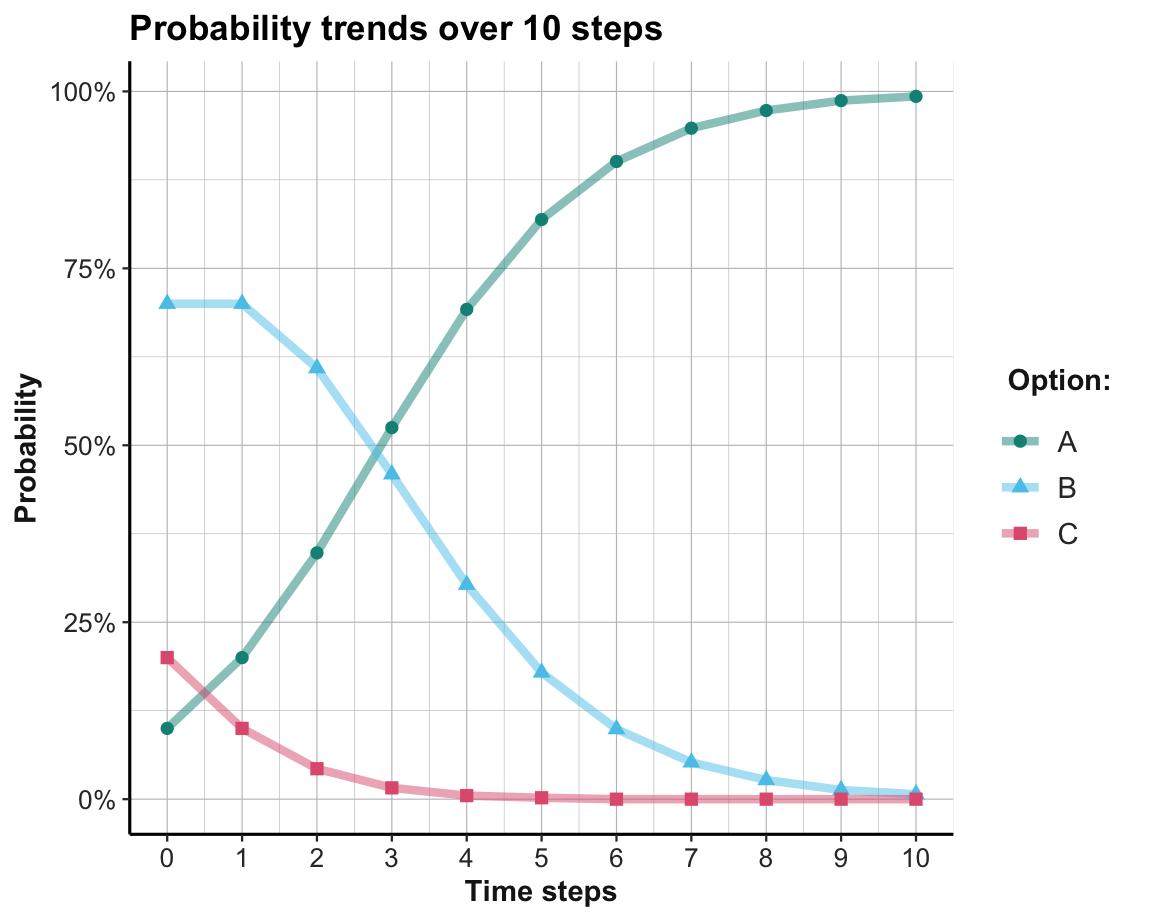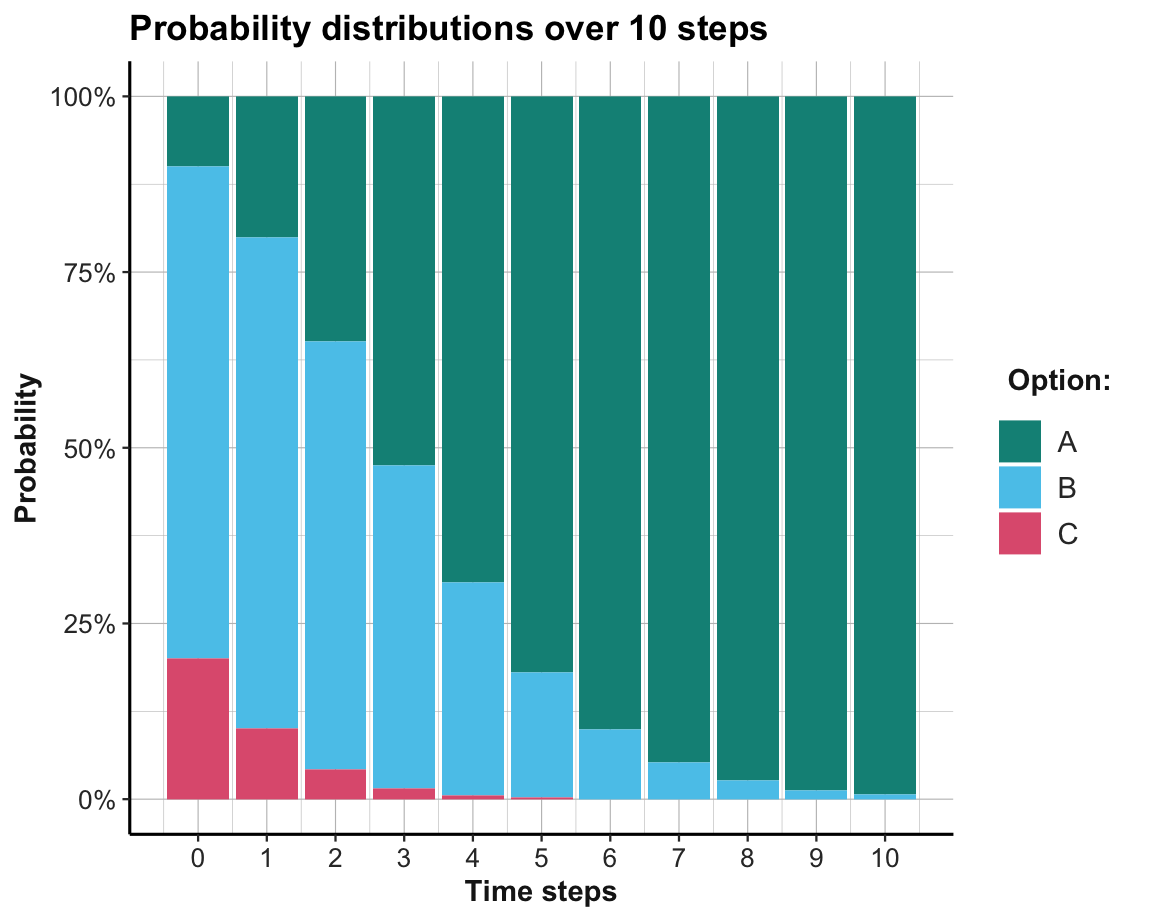## 17.3 Social learning

Learning can be modeled as a function of rewards and the behavior of others. When an option is better than another, it offers higher rewards and will be learned by an agent that explores and exploits an environment to maximize her utility. However, when other agents are present, a second indicator of an option’s quality is its popularity: Other things being equal, better options are more popular.23

### 17.3.1 Replicator dynamics

The basic idea of replicator dynamics (following Page, 2018, p. 308ff.) is simple: The probability of choosing an action is the product of its reward and its popularity.

Given a set of $$N_opt$$ alternatives with corresponding rewards $$\pi(1) ... \pi(n)$$, the probability of choosing an option $$k$$ at time step $$t+1$$ is defined as:

$P_{t+1}(k) = P_{t}(k) \cdot \frac{\pi(k)}{\bar{\pi_{t}}}$

Note that the factor given by the fraction $$\frac{\pi(k)}{\bar{\pi_{t}}}$$ divides the option’s current reward by the current average reward of all options. Its denominator is computed as the sum of all reward values weighted by their probability in the current population. As more popular options are weighted more heavily, this factor combines an effect of reward with an effect of popularity or conformity. Thus, the probability of choosing an option on the next decision cycle depends on its current probability, its current reward, and its current popularity.

Note that this particular conceptualization provides a model for an entire population, rather than any individual element of it. Additionally, the rewards received from each option are assumed to be fixed and independent of the choices, which may be pretty implausible for many real environments.

What changes would signal that the population is learning? The probability distribution of actions being chosen in each time step.

#### Implementation in R

# Environment parameters:
alt  <- c("A", "B", "C")
rew  <- c(20, 10, 5)
prob <- c(.1, .7, .2)  # initial probability distribution
N_t  <- 10  # number of time steps/rounds/trials

# Prepare data storage:
data <- as.data.frame(matrix(NA, ncol = 5, nrow = 10 + 1))
names(data) <- c("t", "avg_rew", paste0("p_", alt))

for (t in 0:N_t){

# (1) Compute average reward:
avg_rew <- sum(prob * rew)

# (+) User feedback/data storage:
# print(paste0(t, ": avg_rew = ", round(avg_rew, 1),
#              ": prob = ", paste0(round(prob, 2), collapse = ":")))
data[(t + 1), ] <- c(t, round(avg_rew, 2), round(prob, 3))

# (2) Update probability:
prob <- prob * rew/avg_rew

}

Given that we collected all intermediate values in data, we can inspect our simulation results by printing the table:

Table 17.3: Data from replicator dynamics.
t avg_rew p_A p_B p_C
0 10.00 0.100 0.700 0.200
1 11.50 0.200 0.700 0.100
2 13.26 0.348 0.609 0.043
3 15.16 0.525 0.459 0.016
4 16.89 0.692 0.303 0.005
5 18.18 0.819 0.179 0.002
6 19.01 0.901 0.099 0.000
7 19.48 0.948 0.052 0.000
8 19.73 0.973 0.027 0.000
9 19.87 0.987 0.013 0.000
10 19.93 0.993 0.007 0.000

As shown in Table 17.3, we initialized our loop at a value of t = 0. This allowed us to include the original situation (prior to any updating of prob) as the first line (i.e., in row data[(t + 1), ]).

Inspecting the rows of Table 17.3 makes it clear that the probabilities of suboptimal options (here: Options B and C) are decreasing, while the probability of choosing the best option (A) is increasing. Thus, options with higher rewards are becoming more popular — and the population quickly converges on choosing the best option.

The population’s systematic shift from poorer to richer options also implies that the value of the average reward (here: avg_rew) is monotonically increasing and approaching the value of the best option. Thus, the function of avg_rew is similar to the role of an aspiration level $$A$$ in reinforcement learning models (see Section 16.2), with the difference that avg_rew reflects the average aspiration of the entire population, whereas $$A_{i}$$ denotes the aspiration level of an individual agent $$i$$.

#### Visualizing results

Ways of depicting the shift in collective dynamics away from bad and towards the best option is provided by the following visualizations of data. Figure 17.4 shows the trends in choosing each option as a function fo the time steps 0 to 10:

library(tidyverse)
library(ds4psy)
library(unikn)

# Re-format data:
data_long <- data %>%
pivot_longer(cols = starts_with("p_"),
names_to = "option",
values_to = "p") %>%
mutate(opt = substr(option, 3, 3))
# data_long

ggplot(data_long, aes(x = t, y = p, group = opt, col = opt)) +
geom_line(size = 1.5, alpha = .5) +
geom_point(aes(shape = opt), size = 2) +
scale_y_continuous(labels = scales::percent_format()) +
scale_x_continuous(breaks = 0:10, labels = 0:10) +
scale_color_manual(values = usecol(c(Seegruen, Seeblau, Pinky))) +
labs(title = paste0("Probability trends over ", N_t, " steps"),
x = "Time steps", y = "Probability",
col = "Option:", shape = "Option:") +
theme_ds4psy()Figure 17.4: Trends in the probabilty of choosing each option per time step.

Note that we re-formatted data into long format prior to plotting (to get the options as one variable, rather than as three separate variables) and changed the y-axis to a percentage scale.

Given that the probability distribution at each time step must sum to 1, it makes sense to display them as a stacked bar chart, with different colors for each option (see Figure 17.5):

ggplot(data_long, aes(x = t, y = p, fill = opt)) +
geom_bar(position = "fill", stat = "identity") +
scale_y_continuous(labels = scales::percent_format()) +
scale_x_continuous(breaks = 0:10, labels = 0:10) +
scale_fill_manual(values = usecol(c(Seegruen, Seeblau, Pinky))) +
labs(title = paste0("Probability distributions over ", N_t, " steps"),
x = "Time steps", y = "Probability",
col = "Option:", fill = "Option:") +
theme_ds4psy()Figure 17.5: The probabilty of choosing options per time step.

This shows that the best option (here: Option A) is unpopular at first, but becomes the dominant one by about the 4-th time step. The learning process shown here appears to be much quicker than that of an individual reinforcement learner (in Section 16.2). This is mostly due to a change in our level of analysis: Our model of replicator dynamics describes an entire population of agents. In fact, our use of the probability distribution as a proxy for the popularity of options implicitly assumes that an infinite population of agents is experiencing the environment and both exploring and exploiting the full set of all options on every time step. Whereas an individual RL agent must first explore options and — if it gets unlucky — waste a lot of valuable time on inferior options, a population of agents can evaluate the entire option range and rapidly converge on the best option.

Convergence on the best option is guaranteed as long all options are chosen (i.e., have an initial probability of $$p_{t=0}(i)>0$$) and the population of agents is large (ideally infinite).

#### Practice

1. Answer the following questions by studying the basic equation of replicator dynamics:

• What’s the impact of options that offer zero rewards (i.e, $$\pi(k)=0$$)?

• What’s the impact of options that are not chosen (i.e, $$P_{t}(k)=0$$)?

2. What happens if the three options (A–C) yield identical rewards (e.g., 10 units for each option)?

3. What happens if the rewards of three options (A–C) yield rewards of 10, 20, and 70 units, but their initial popularities are reversed (i.e., 70%, 20%, 10%).

• Do 10 time steps still suffice to learn the best option?

• What if the initial contrast is even more extreme, with rewards of 1, 2, and 97 units, and initial probabilities of 97%, 2%, and 1%, respectively?

Hint: Run these simulations in your mind first, then check your predictions with the code above.

1. What real-world environment could qualify as

• one in which the rewards of all objects are stable and independent of the agents’ actions?

• one in which all agents also have identical preferences?

1. How would the learning process change

• if the number of agents was discrete (e.g., 10)?

• if the number of options exceeded the number of agents?

Hint: Consider the range of values that prob would and could take in both cases.

### References

Page, S. E. (2018). The model thinker: What you need to know to make data work for you. Basic Books.

1. The list of “other things” that are assumed to be equal here is pretty long (e.g., including some stability in options and preferences).↩︎